+关注继续查看

# 图像和视频分析

## 一、Python OpenCV 入门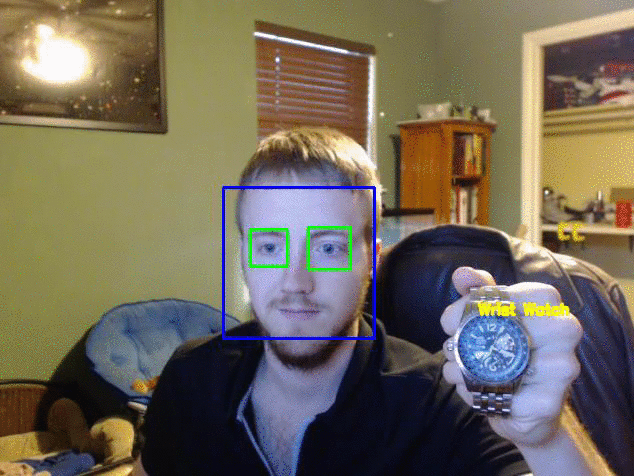### Windows 用户：

python-OpenCV：有其他的方法，但这是最简单的。 下载相应的 wheel（.whl）文件，然后使用pip进行安装。 观看视频来寻求帮助。

pip install numpy

pip install matplotlib

### Linux/Mac 用户

pip3 install numpy 

apt-get install python3-numpy

pip3 install matplotlib 

apt-get install python3-matplotlib

apt-get install python-OpenCV

Matplotlib 是用于展示来自视频或图像的帧的可选选项。 我们将在这里展示几个使用它的例子。 Numpy 被用于“数值和 Python”的所有东西。 我们主要利用 Numpy 的数组功能。 最后，我们使用python-OpenCV，它是 Python 特定的 OpenCV 绑定。

OpenCV 有一些操作，如果没有完整安装 OpenCV （大小约 3GB），你将无法完成，但是实际上你可以用 python-OpenCV 最简安装。 我们将在本系列的后续部分中使用 OpenCV 的完整安装，如果你愿意的话，你可以随意获得它，但这三个模块将使我们忙碌一段时间！

import cv2
import matplotlib
import numpy

### 背景提取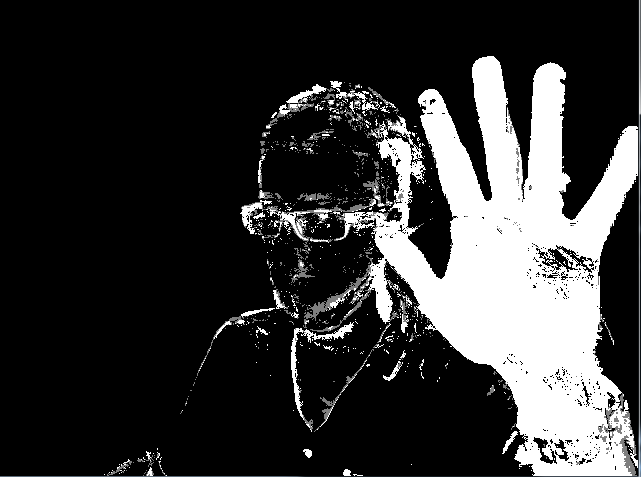### 颜色过滤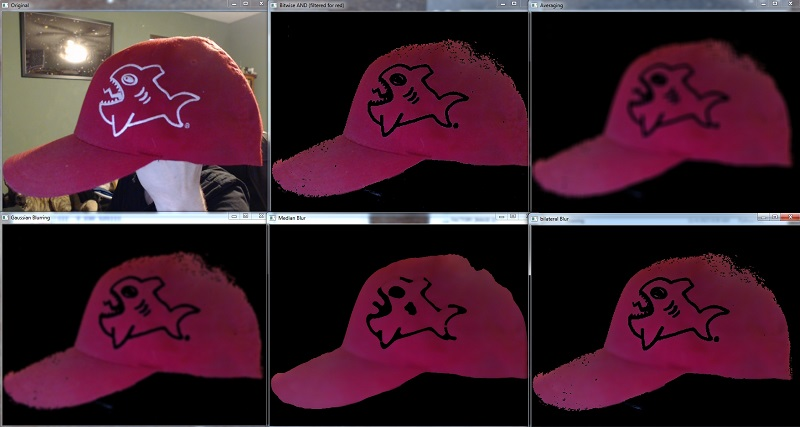### 边缘检测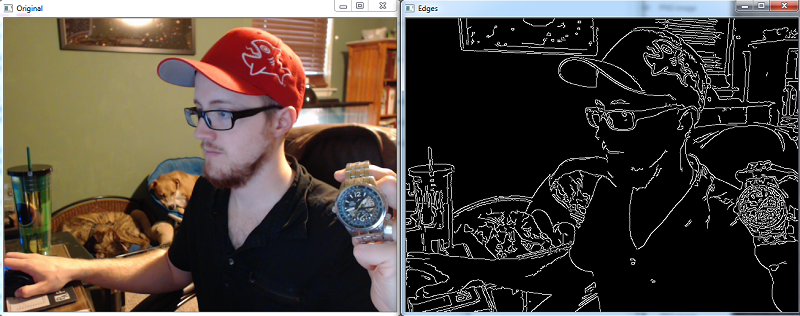### 用于对象识别的特征匹配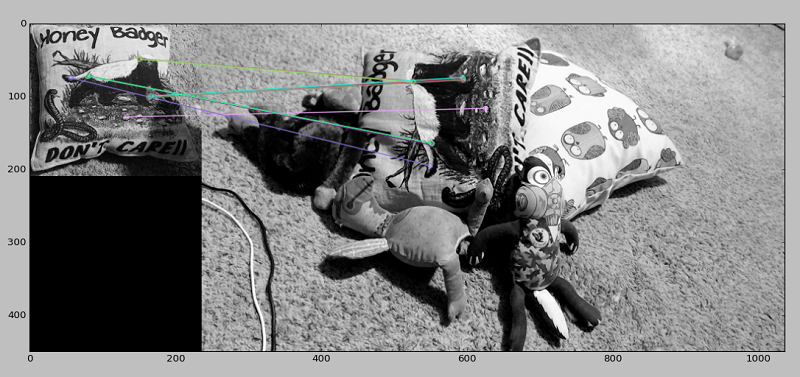### 一般对象识别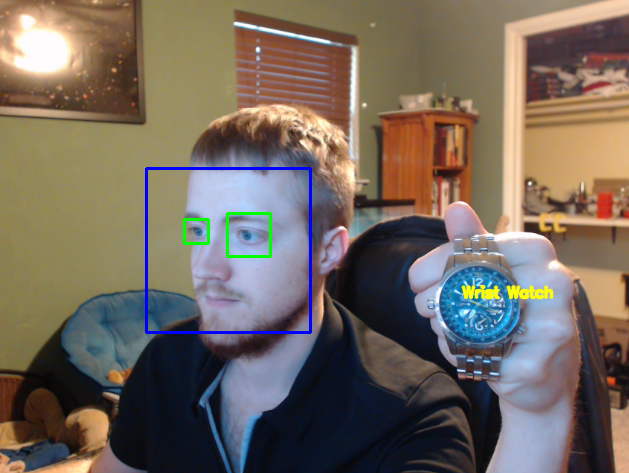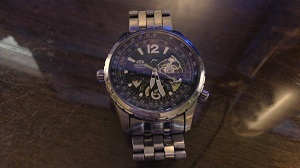import cv2
import numpy as np
from matplotlib import pyplot as plt

cv2.imshow('image',img)
cv2.waitKey(0)
cv2.destroyAllWindows()

import cv2
import numpy as np
from matplotlib import pyplot as plt

plt.imshow(img, cmap = 'gray', interpolation = 'bicubic')
plt.xticks([]), plt.yticks([])  # to hide tick values on X and Y axis
plt.plot([200,300,400],[100,200,300],'c', linewidth=5)
plt.show()

cv2.imwrite('watchgray.png',img)

## 二、加载视频源

import numpy as np
import cv2

cap = cv2.VideoCapture(0)

while(True):
gray = cv2.cvtColor(frame, cv2.COLOR_BGR2GRAY)

cv2.imshow('frame',gray)
if cv2.waitKey(1) & 0xFF == ord('q'):
break

cap.release()
cv2.destroyAllWindows()

while(True):
ret, frame = cap.read()

    gray = cv2.cvtColor(frame, cv2.COLOR_BGR2GRAY)

    cv2.imshow('frame',gray)

    if cv2.waitKey(1) & 0xFF == ord('q'):
break

cap.release()
cv2.destroyAllWindows()

import numpy as np
import cv2

cap = cv2.VideoCapture(1)
fourcc = cv2.VideoWriter_fourcc(*'XVID')
out = cv2.VideoWriter('output.avi',fourcc, 20.0, (640,480))

while(True):
gray = cv2.cvtColor(frame, cv2.COLOR_BGR2GRAY)
out.write(frame)
cv2.imshow('frame',gray)
if cv2.waitKey(1) & 0xFF == ord('q'):
break

cap.release()
out.release()
cv2.destroyAllWindows()

## 三、在图像上绘制和写字import numpy as np
import cv2

img = cv2.imread('watch.jpg',cv2.IMREAD_COLOR)

cv2.line(img,(0,0),(150,150),(255,255,255),15)

cv2.imshow('image',img)
cv2.waitKey(0)
cv2.destroyAllWindows()

cv2.line()接受以下参数：图片，开始坐标，结束坐标，颜色（bgr），线条粗细。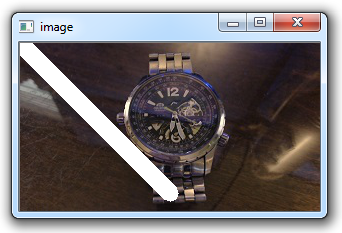cv2.rectangle(img,(15,25),(200,150),(0,0,255),15)

cv2.circle(img,(100,63), 55, (0,255,0), -1)

pts = np.array([[10,5],[20,30],[70,20],[50,10]], np.int32)
# OpenCV documentation had this code, which reshapes the array to a 1 x 2. I did not
# find this necessary, but you may:
#pts = pts.reshape((-1,1,2))
cv2.polylines(img, [pts], True, (0,255,255), 3)

font = cv2.FONT_HERSHEY_SIMPLEX
cv2.putText(img,'OpenCV Tuts!',(0,130), font, 1, (200,255,155), 2, cv2.LINE_AA)

import numpy as np
import cv2

cv2.line(img,(0,0),(200,300),(255,255,255),50)
cv2.rectangle(img,(500,250),(1000,500),(0,0,255),15)
cv2.circle(img,(447,63), 63, (0,255,0), -1)
pts = np.array([[100,50],[200,300],[700,200],[500,100]], np.int32)
pts = pts.reshape((-1,1,2))
cv2.polylines(img, [pts], True, (0,255,255), 3)
font = cv2.FONT_HERSHEY_SIMPLEX
cv2.putText(img,'OpenCV Tuts!',(10,500), font, 6, (200,255,155), 13, cv2.LINE_AA)
cv2.imshow('image',img)
cv2.waitKey(0)
cv2.destroyAllWindows()## 四、图像操作import cv2
import numpy as np

img = cv2.imread('watch.jpg',cv2.IMREAD_COLOR)

px = img[55,55]

img[55,55] = [255,255,255]

px = img[55,55]
print(px)

px = img[100:150,100:150]
print(px)

img[100:150,100:150] = [255,255,255]

print(img.shape)
print(img.size)
print(img.dtype)

watch_face = img[37:111,107:194]
img[0:74,0:87] = watch_face

cv2.imshow('image',img)
cv2.waitKey(0)
cv2.destroyAllWindows()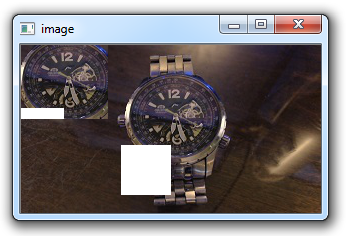## 五、图像算术和逻辑运算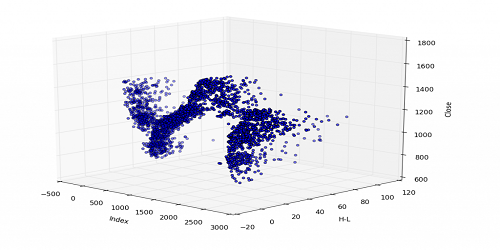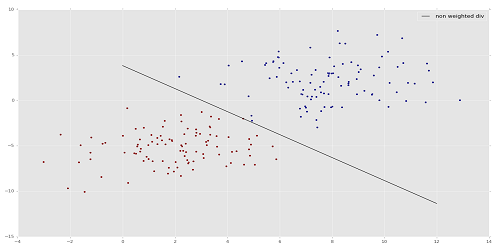import cv2
import numpy as np

# 500 x 250

cv2.waitKey(0)
cv2.destroyAllWindows()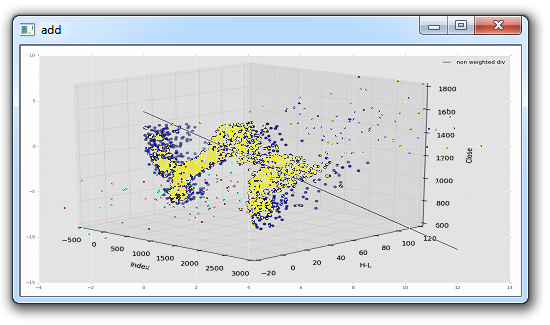add = cv2.add(img1,img2)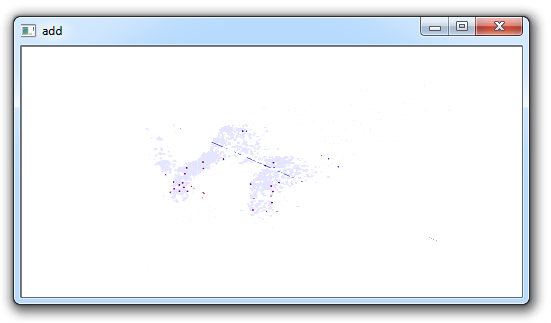import cv2
import numpy as np

weighted = cv2.addWeighted(img1, 0.6, img2, 0.4, 0)
cv2.imshow('weighted',weighted)
cv2.waitKey(0)
cv2.destroyAllWindows()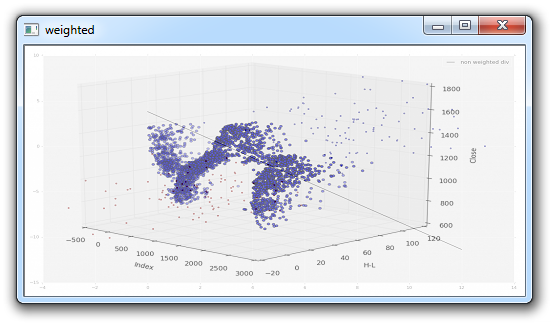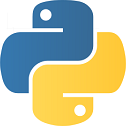import cv2
import numpy as np

# I want to put logo on top-left corner, So I create a ROI
rows,cols,channels = img2.shape
roi = img1[0:rows, 0:cols ]

# Now create a mask of logo and create its inverse mask
img2gray = cv2.cvtColor(img2,cv2.COLOR_BGR2GRAY)

ret, mask = cv2.threshold(img2gray, 220, 255, cv2.THRESH_BINARY_INV)

# Now black-out the area of logo in ROI

# Take only region of logo from logo image.

img1[0:rows, 0:cols ] = dst

cv2.imshow('res',img1)
cv2.waitKey(0)
cv2.destroyAllWindows()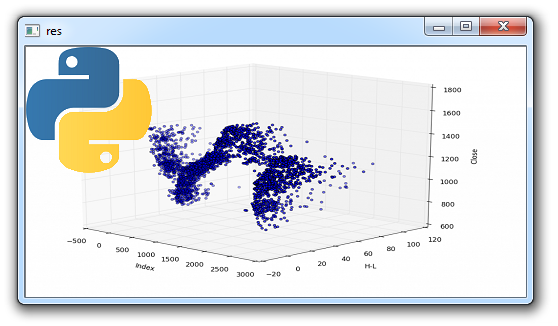## 六、阈值retval, threshold = cv2.threshold(img, 10, 255, cv2.THRESH_BINARY)

import cv2
import numpy as np

retval, threshold = cv2.threshold(img, 12, 255, cv2.THRESH_BINARY)
cv2.imshow('original',img)
cv2.imshow('threshold',threshold)
cv2.waitKey(0)
cv2.destroyAllWindows()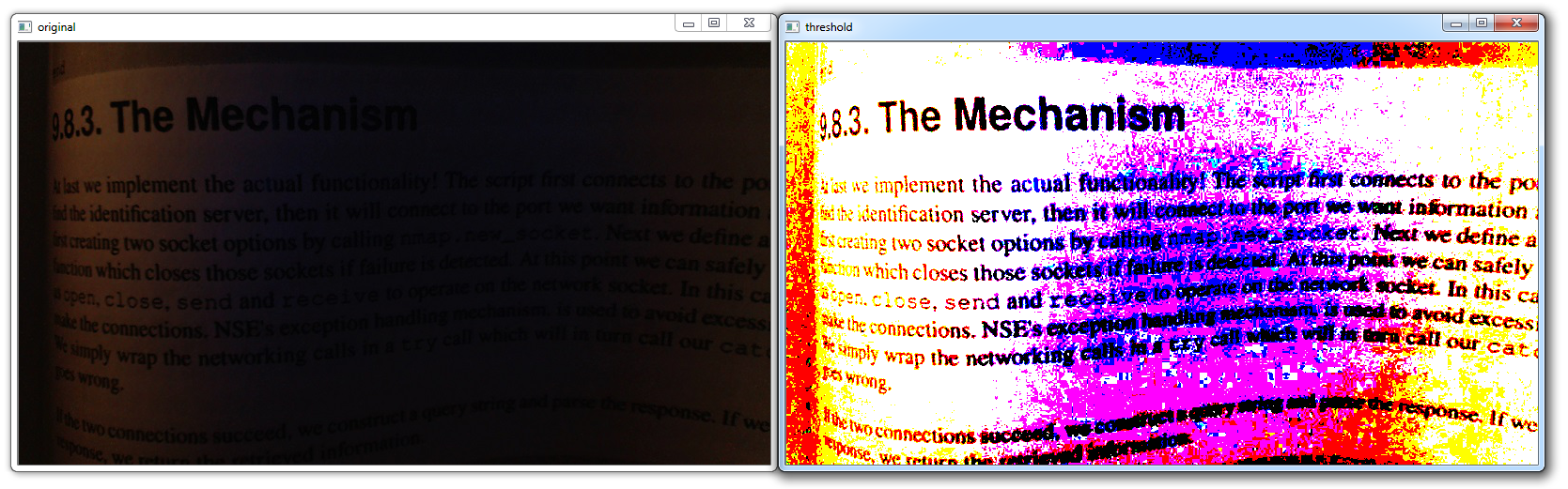import cv2
import numpy as np

grayscaled = cv2.cvtColor(img,cv2.COLOR_BGR2GRAY)
retval, threshold = cv2.threshold(grayscaled, 10, 255, cv2.THRESH_BINARY)
cv2.imshow('original',img)
cv2.imshow('threshold',threshold)
cv2.waitKey(0)
cv2.destroyAllWindows()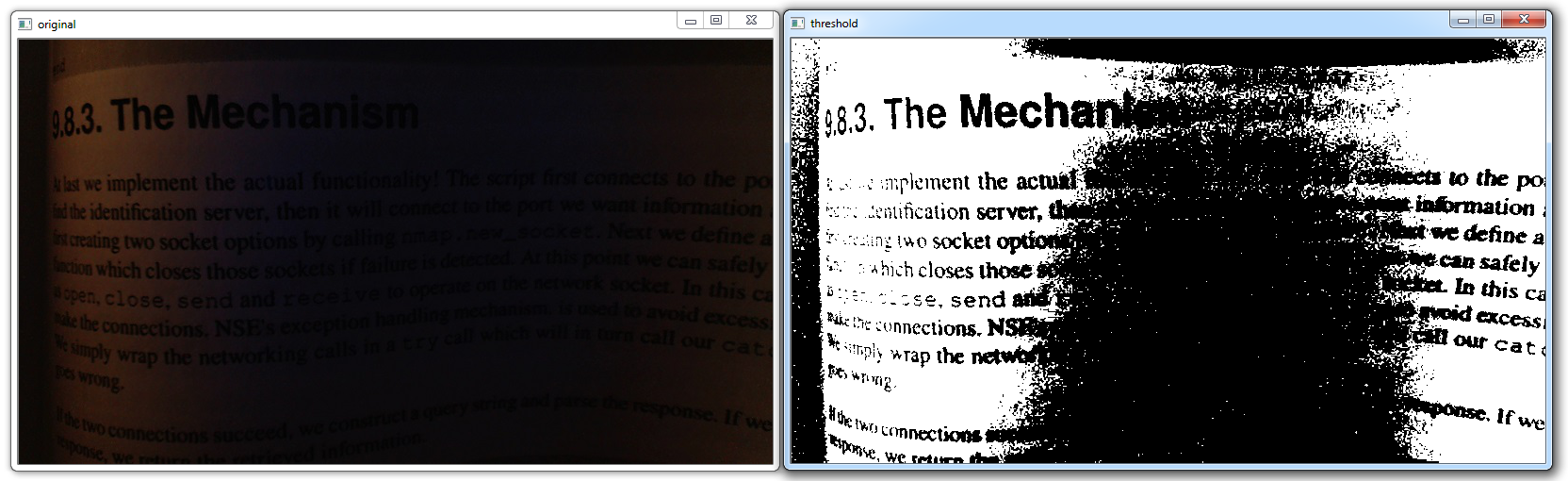import cv2
import numpy as np

cv2.imshow('original',img)
cv2.waitKey(0)
cv2.destroyAllWindows()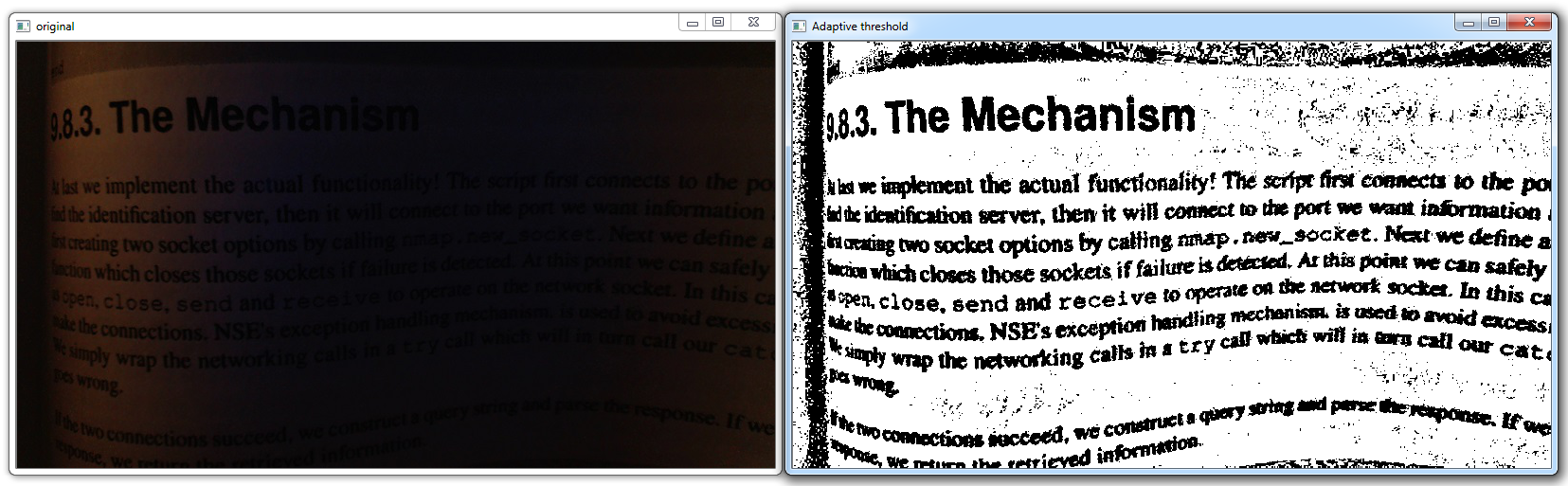retval2,threshold2 = cv2.threshold(grayscaled,125,255,cv2.THRESH_BINARY+cv2.THRESH_OTSU)
cv2.imshow('original',img)
cv2.imshow('Otsu threshold',threshold2)
cv2.waitKey(0)
cv2.destroyAllWindows()

## 七、颜色过滤

import cv2
import numpy as np

cap = cv2.VideoCapture(0)

while(1):
hsv = cv2.cvtColor(frame, cv2.COLOR_BGR2HSV)

lower_red = np.array([30,150,50])
upper_red = np.array([255,255,180])

cv2.imshow('frame',frame)
cv2.imshow('res',res)

k = cv2.waitKey(5) & 0xFF
if k == 27:
break

cv2.destroyAllWindows()
cap.release()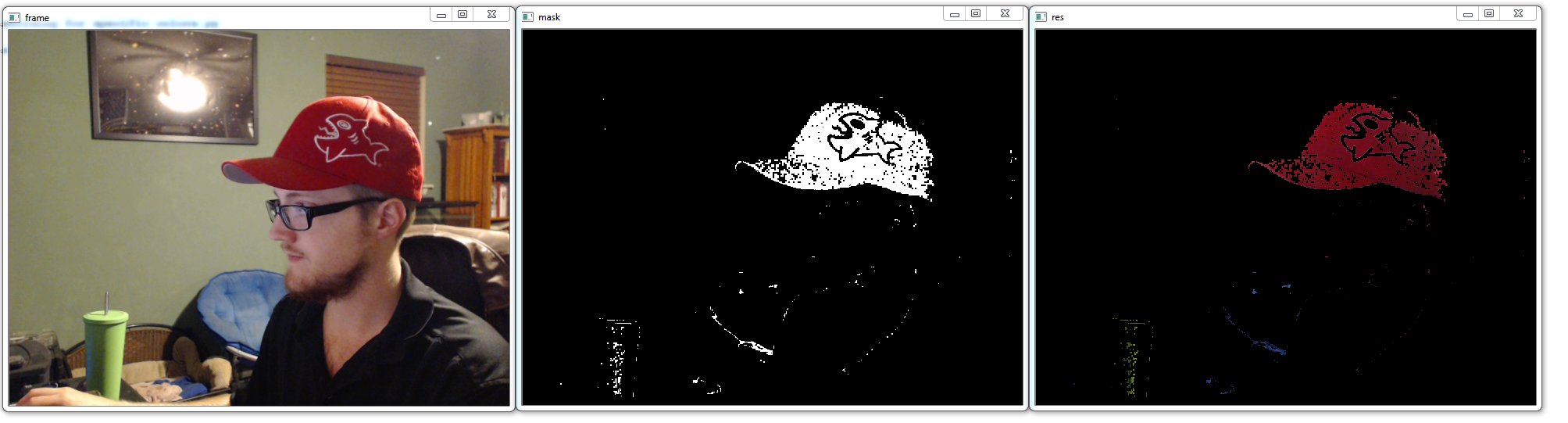dark_red  = np.uint8([[[12,22,121]]])
dark_red = cv2.cvtColor(dark_red,cv2.COLOR_BGR2HSV)

## 八、模糊和平滑import cv2
import numpy as np

cap = cv2.VideoCapture(0)

while(1):

hsv = cv2.cvtColor(frame, cv2.COLOR_BGR2HSV)

lower_red = np.array([30,150,50])
upper_red = np.array([255,255,180])

res = cv2.bitwise_and(frame,frame, mask= mask)

    kernel = np.ones((15,15),np.float32)/225
smoothed = cv2.filter2D(res,-1,kernel)
cv2.imshow('Original',frame)
cv2.imshow('Averaging',smoothed)

k = cv2.waitKey(5) & 0xFF
if k == 27:
break

cv2.destroyAllWindows()
cap.release()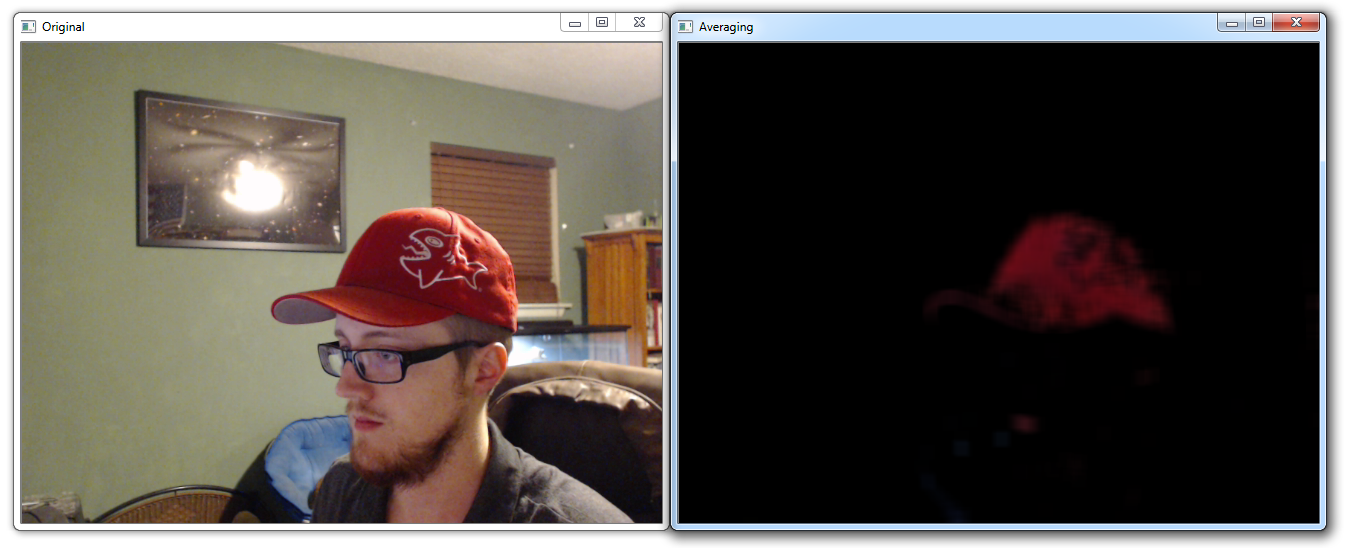blur = cv2.GaussianBlur(res,(15,15),0)
cv2.imshow('Gaussian Blurring',blur)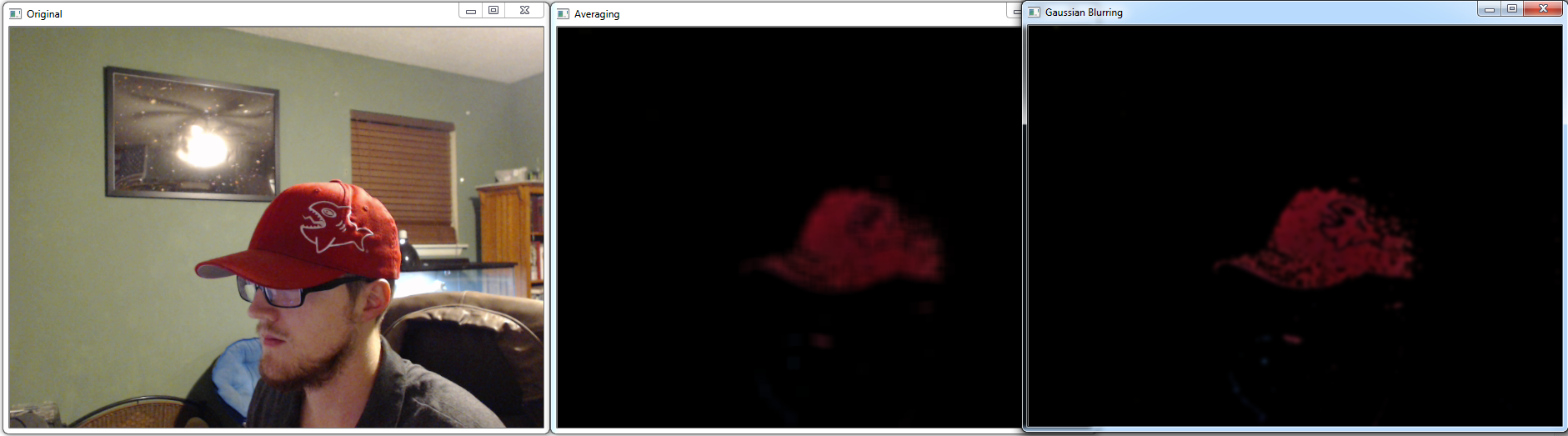median = cv2.medianBlur(res,15)
cv2.imshow('Median Blur',median)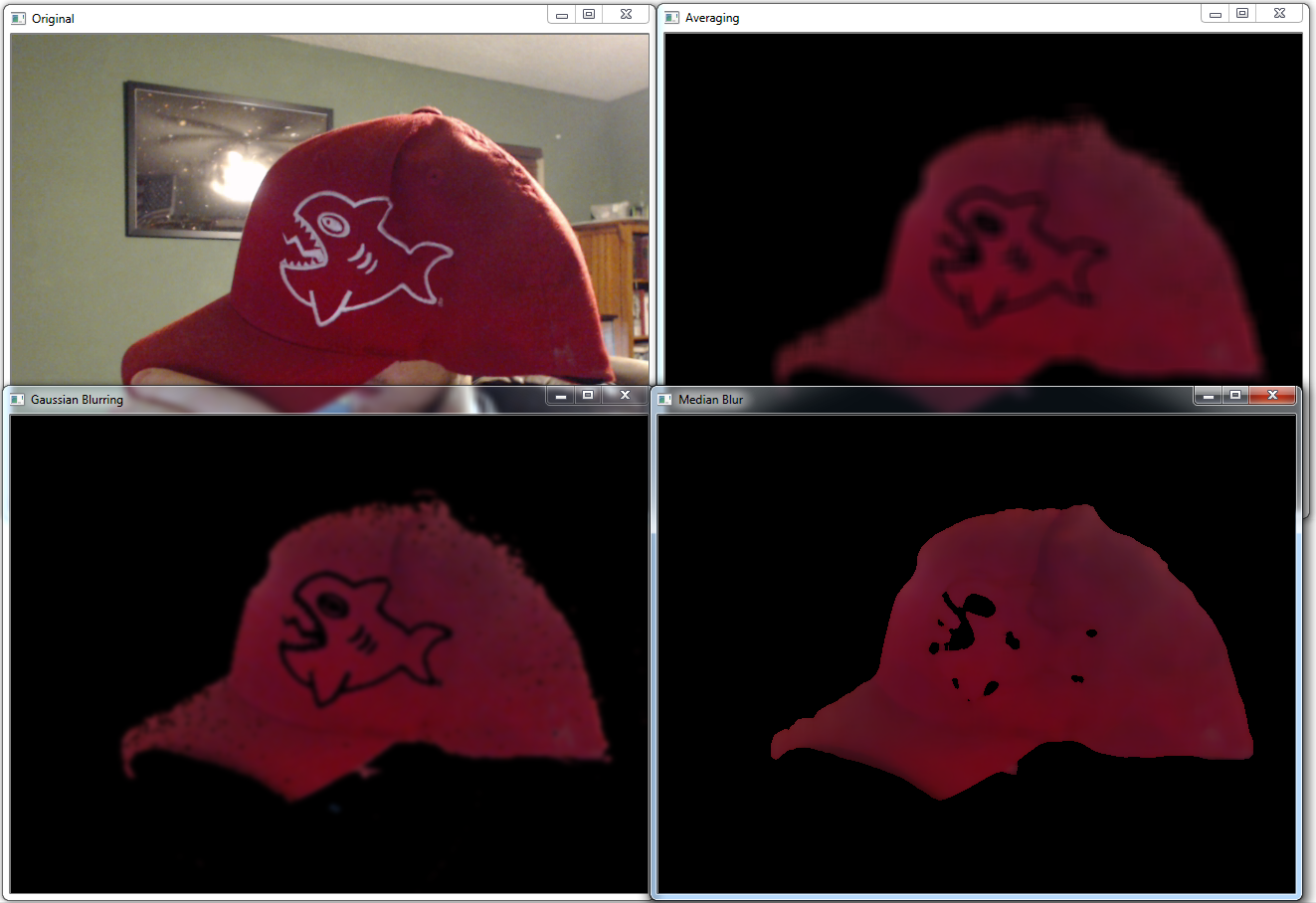bilateral = cv2.bilateralFilter(res,15,75,75)
cv2.imshow('bilateral Blur',bilateral)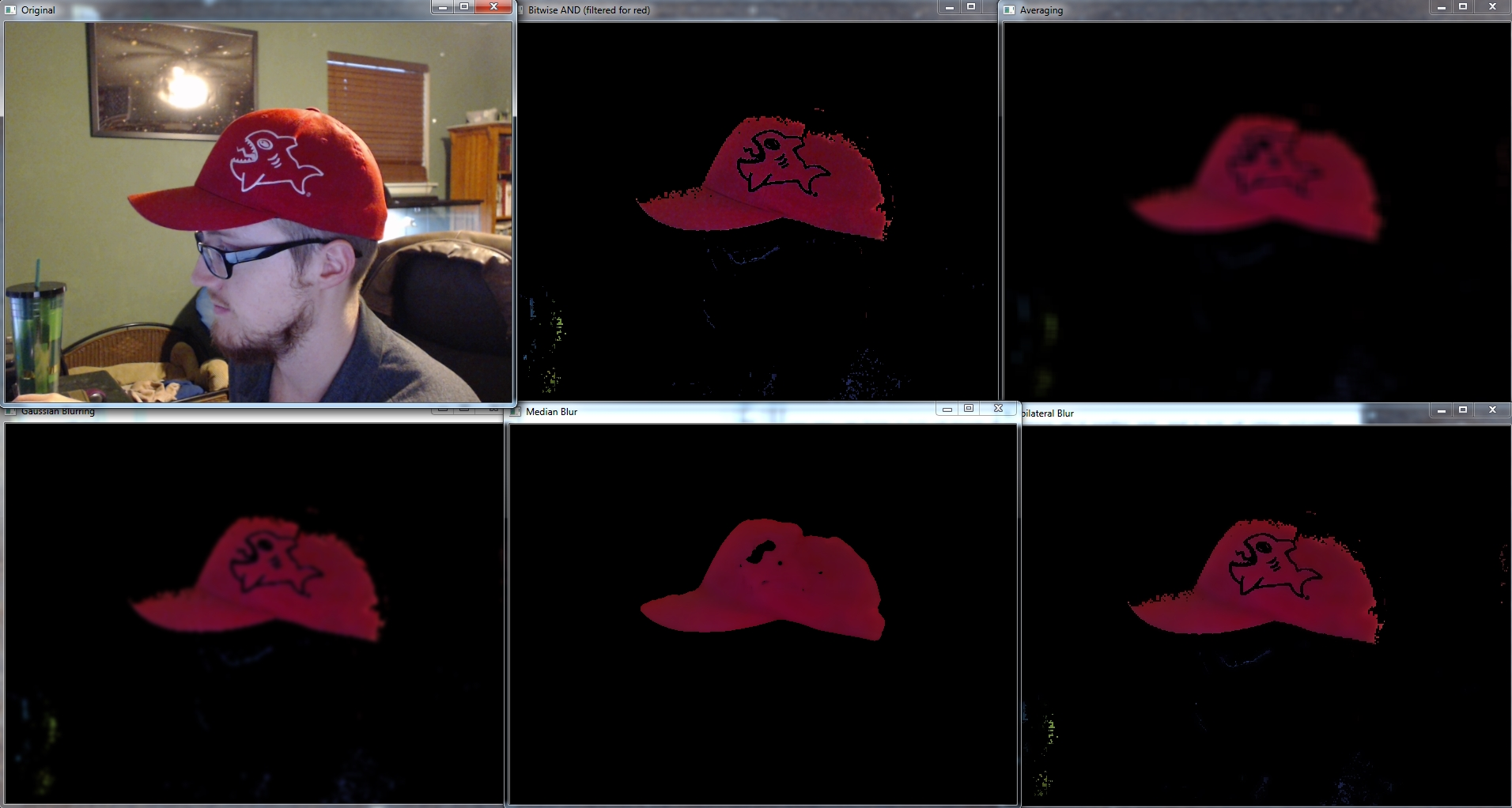## 九、形态变换

import cv2
import numpy as np

cap = cv2.VideoCapture(0)

while(1):

hsv = cv2.cvtColor(frame, cv2.COLOR_BGR2HSV)

lower_red = np.array([30,150,50])
upper_red = np.array([255,255,180])

kernel = np.ones((5,5),np.uint8)

cv2.imshow('Original',frame)
cv2.imshow('Erosion',erosion)
cv2.imshow('Dilation',dilation)

k = cv2.waitKey(5) & 0xFF
if k == 27:
break

cv2.destroyAllWindows()
cap.release()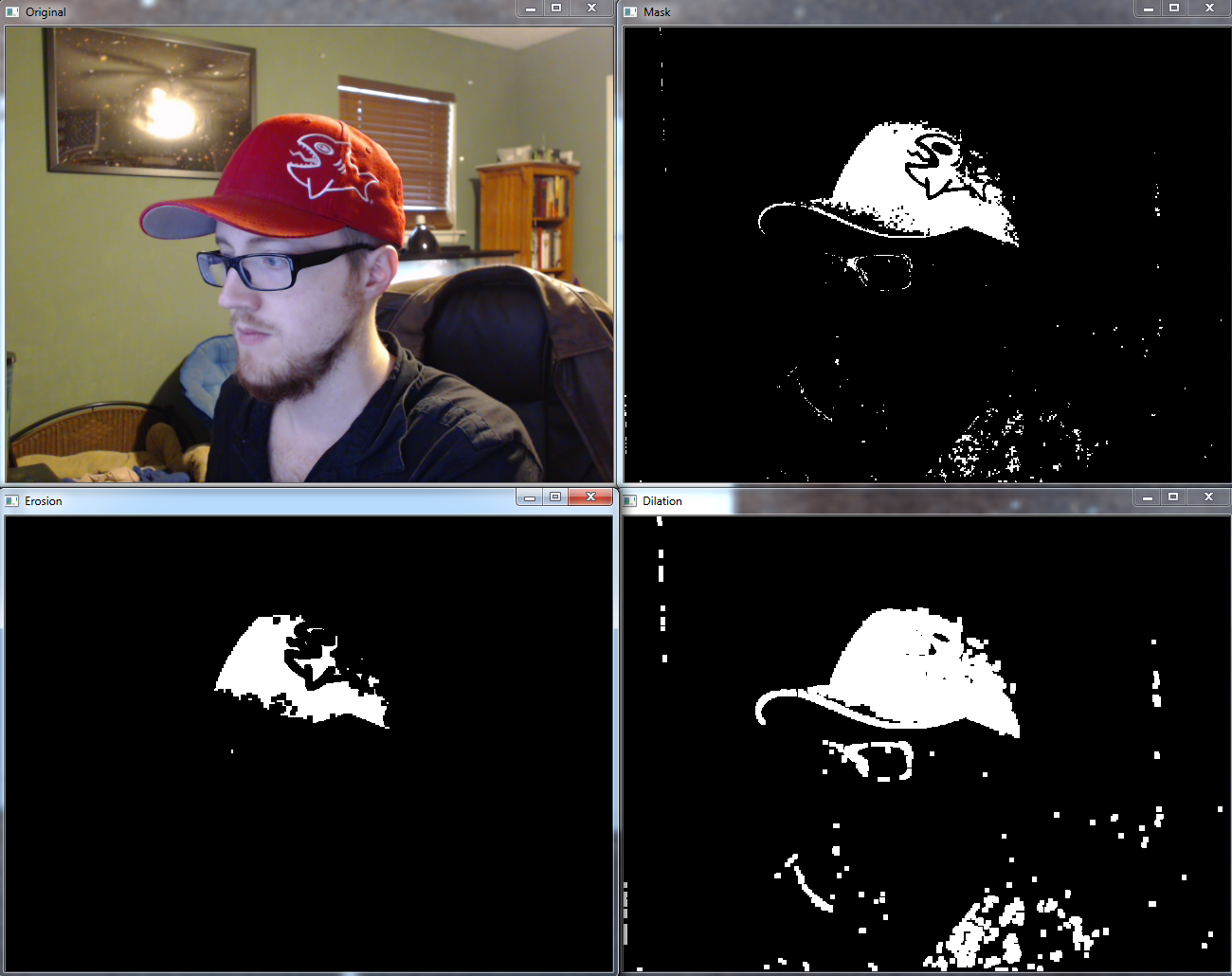cap = cv2.VideoCapture(1)

while(1):

hsv = cv2.cvtColor(frame, cv2.COLOR_BGR2HSV)

lower_red = np.array([30,150,50])
upper_red = np.array([255,255,180])

kernel = np.ones((5,5),np.uint8)

cv2.imshow('Original',frame)
cv2.imshow('Opening',opening)
cv2.imshow('Closing',closing)

k = cv2.waitKey(5) & 0xFF
if k == 27:
break

cv2.destroyAllWindows()
cap.release()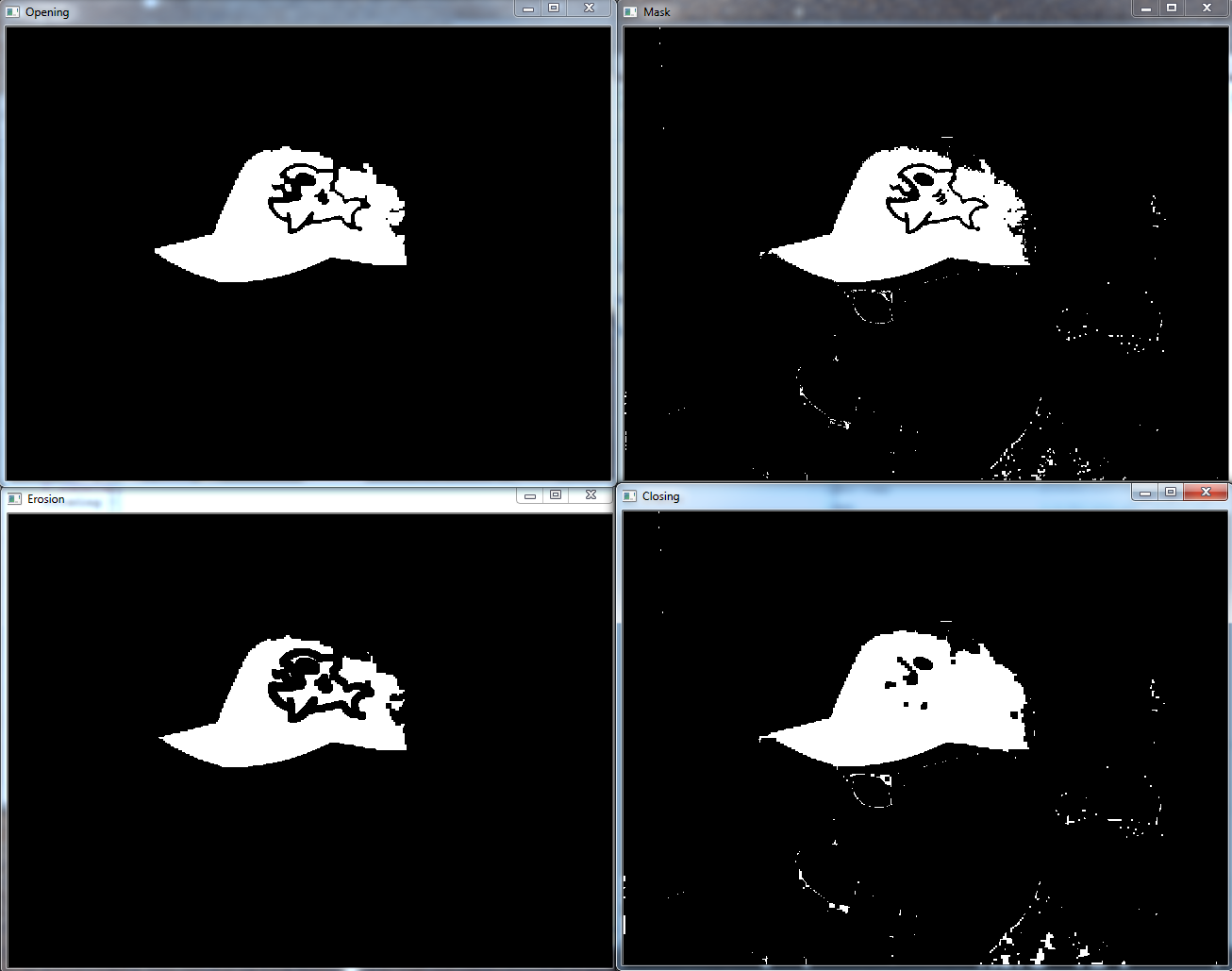# It is the difference between input image and Opening of the image
cv2.imshow('Tophat',tophat)

# It is the difference between the closing of the input image and input image.
cv2.imshow('Blackhat',blackhat)

## 十、边缘检测和渐变

import cv2
import numpy as np

cap = cv2.VideoCapture(1)

while(1):

# Take each frame
hsv = cv2.cvtColor(frame, cv2.COLOR_BGR2HSV)

lower_red = np.array([30,150,50])
upper_red = np.array([255,255,180])

laplacian = cv2.Laplacian(frame,cv2.CV_64F)
sobelx = cv2.Sobel(frame,cv2.CV_64F,1,0,ksize=5)
sobely = cv2.Sobel(frame,cv2.CV_64F,0,1,ksize=5)

cv2.imshow('Original',frame)
cv2.imshow('laplacian',laplacian)
cv2.imshow('sobelx',sobelx)
cv2.imshow('sobely',sobely)

k = cv2.waitKey(5) & 0xFF
if k == 27:
break

cv2.destroyAllWindows()
cap.release()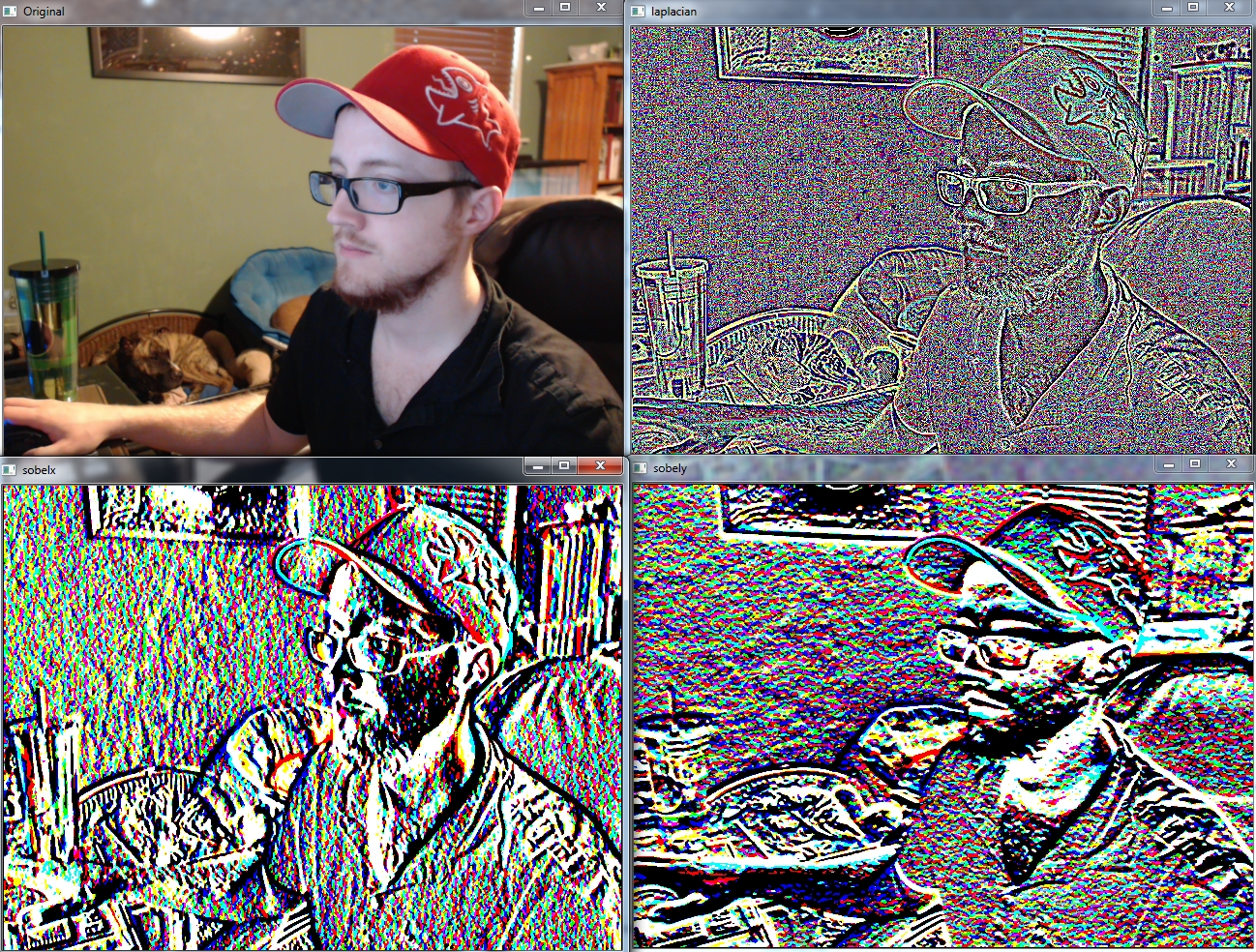import cv2
import numpy as np

cap = cv2.VideoCapture(0)

while(1):

hsv = cv2.cvtColor(frame, cv2.COLOR_BGR2HSV)

lower_red = np.array([30,150,50])
upper_red = np.array([255,255,180])

cv2.imshow('Original',frame)
edges = cv2.Canny(frame,100,200)
cv2.imshow('Edges',edges)

k = cv2.waitKey(5) & 0xFF
if k == 27:
break

cv2.destroyAllWindows()
cap.release()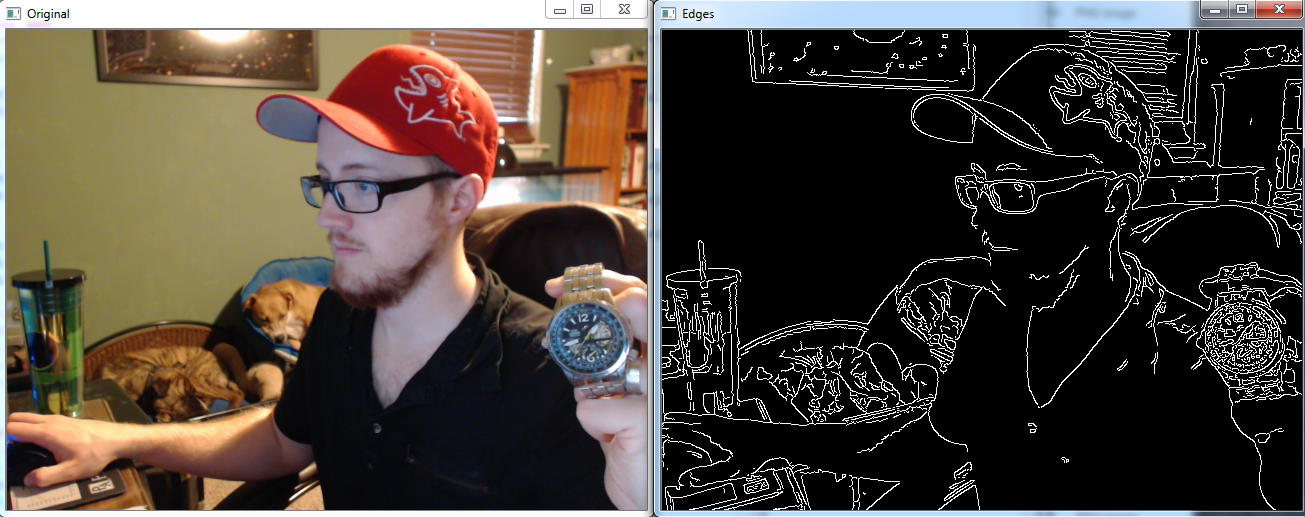## 十一、模板匹配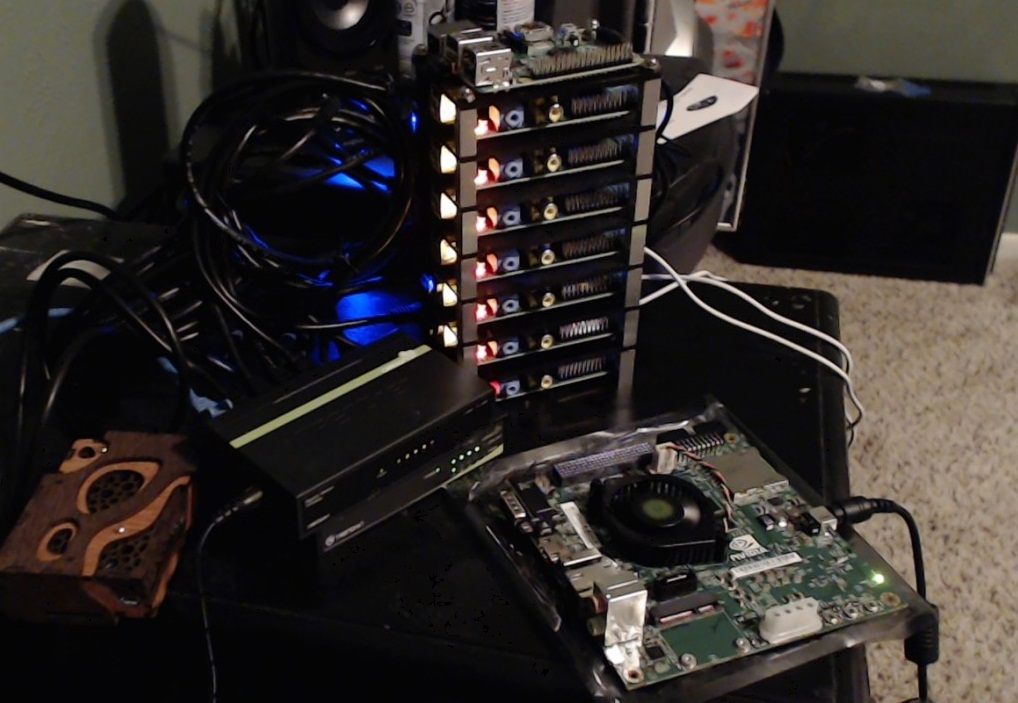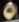import cv2
import numpy as np

img_gray = cv2.cvtColor(img_rgb, cv2.COLOR_BGR2GRAY)

w, h = template.shape[::-1]

res = cv2.matchTemplate(img_gray,template,cv2.TM_CCOEFF_NORMED)
threshold = 0.8
loc = np.where( res >= threshold)

for pt in zip(*loc[::-1]):
cv2.rectangle(img_rgb, pt, (pt + w, pt + h), (0,255,255), 2)

cv2.imshow('Detected',img_rgb)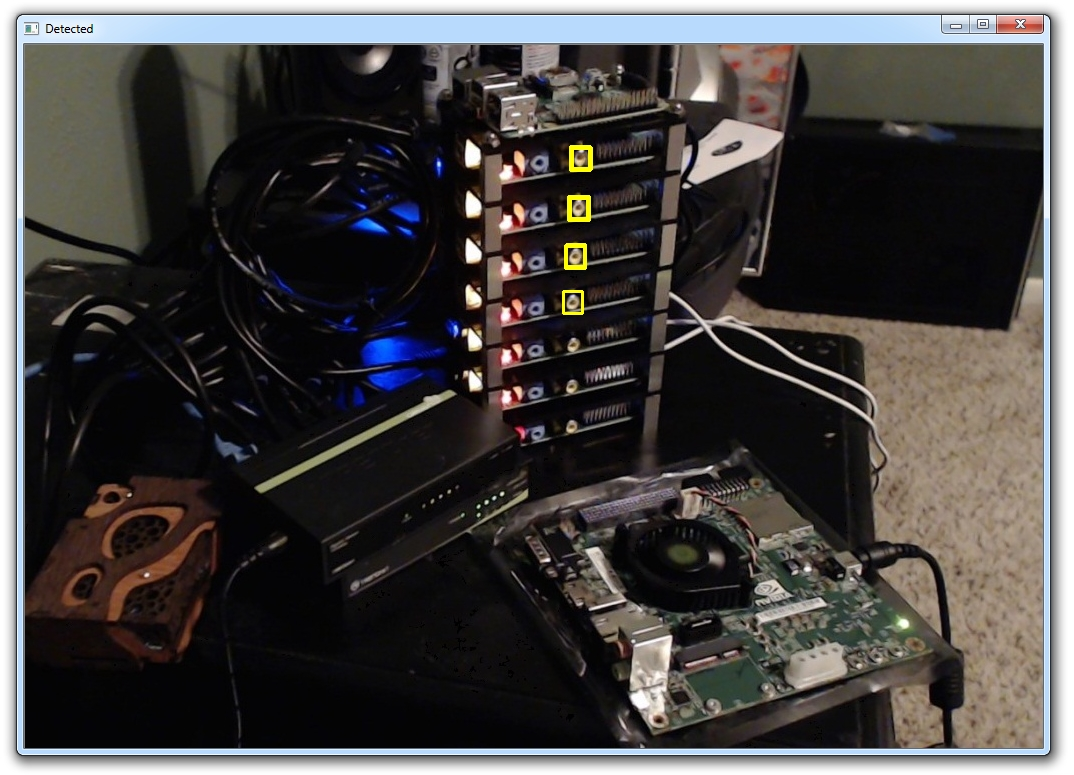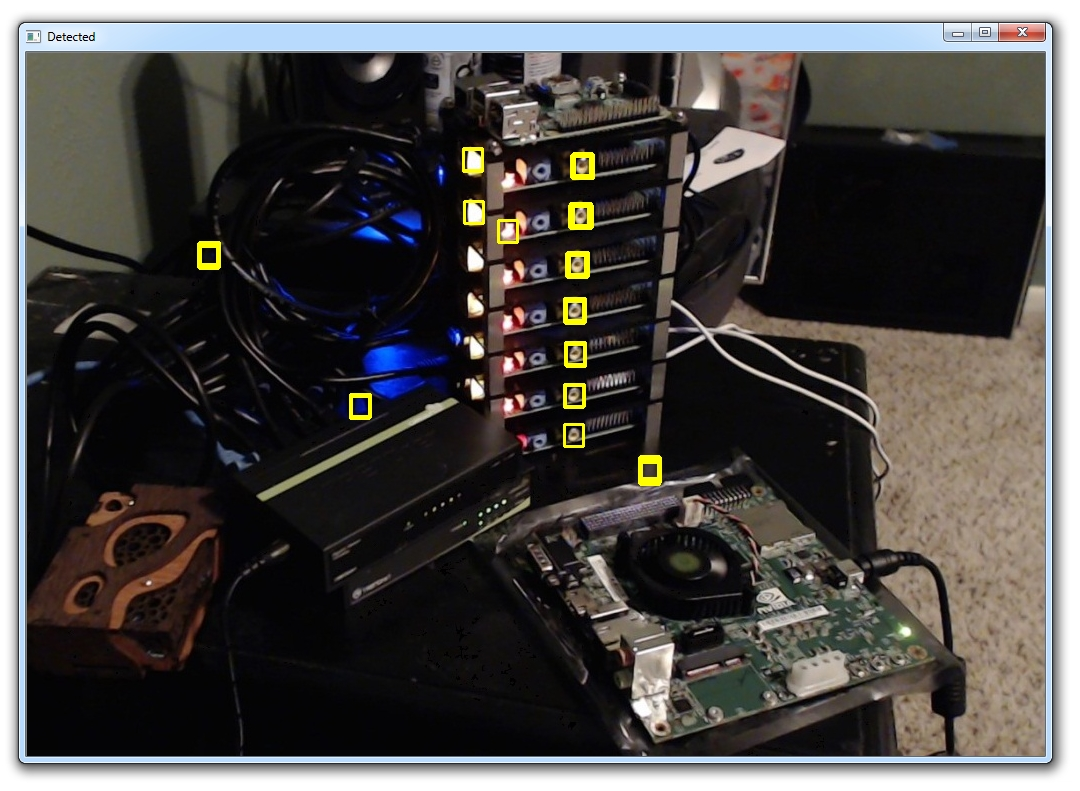## 十二、GrabCut 前景提取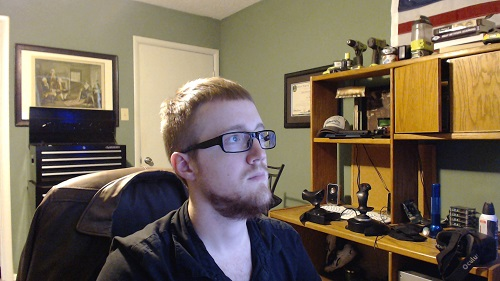import numpy as np
import cv2
from matplotlib import pyplot as plt

bgdModel = np.zeros((1,65),np.float64)
fgdModel = np.zeros((1,65),np.float64)

rect = (161,79,150,150)

cv2.grabCut(img,mask,rect,bgdModel,fgdModel,5,cv2.GC_INIT_WITH_RECT)

plt.imshow(img)
plt.colorbar()
plt.show()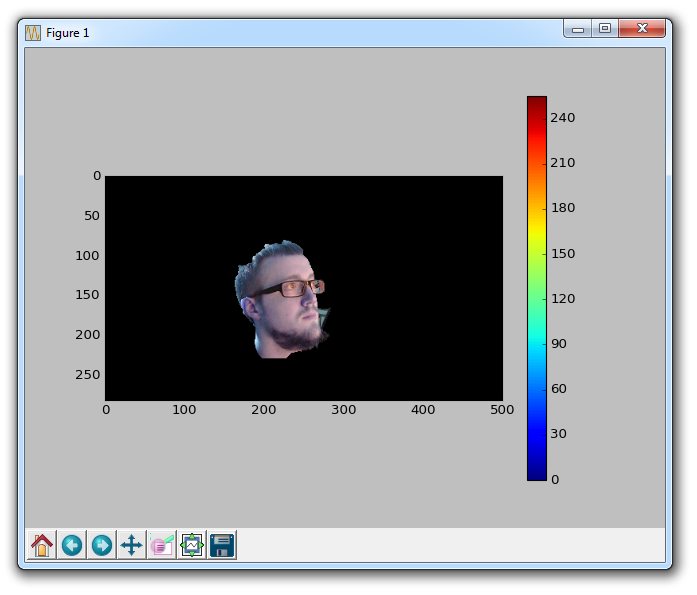## 十三、角点检测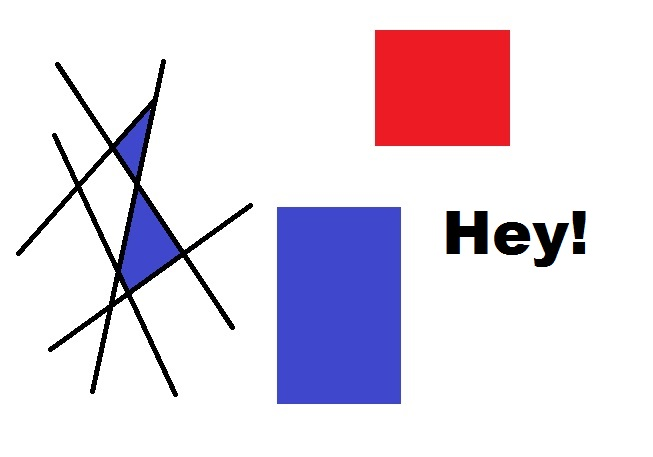import numpy as np
import cv2

gray = cv2.cvtColor(img,cv2.COLOR_BGR2GRAY)
gray = np.float32(gray)

corners = cv2.goodFeaturesToTrack(gray, 100, 0.01, 10)
corners = np.int0(corners)

for corner in corners:
x,y = corner.ravel()
cv2.circle(img,(x,y),3,255,-1)

cv2.imshow('Corner',img)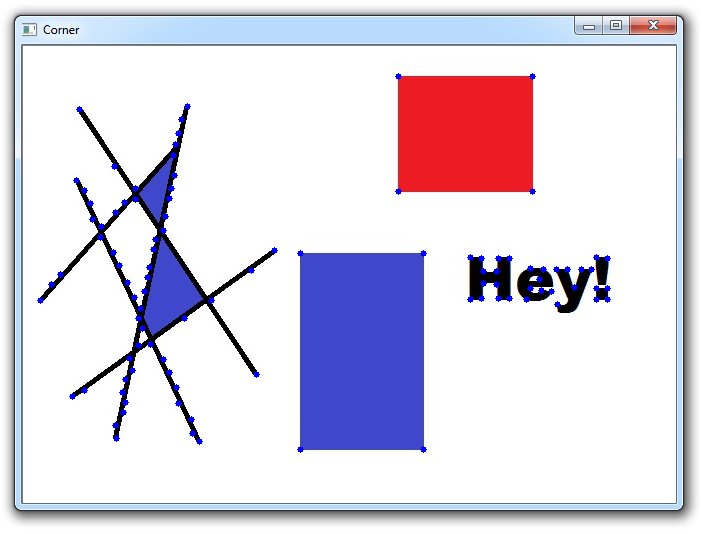## 十四、特征匹配（单映射）爆破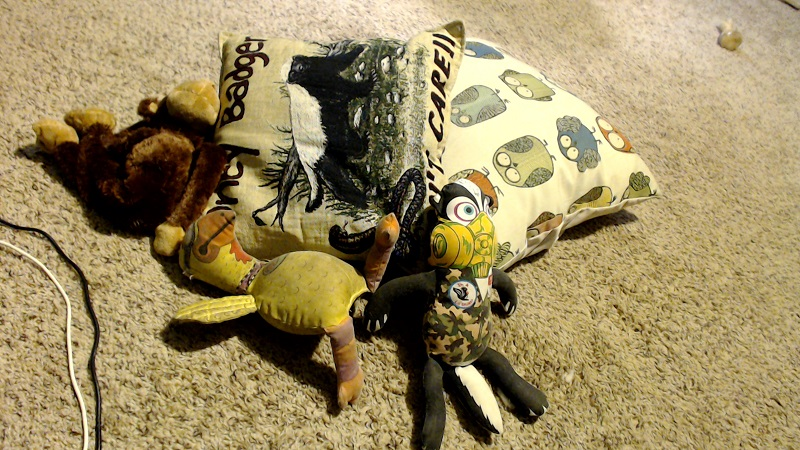import numpy as np
import cv2
import matplotlib.pyplot as plt

img2 = cv2.imread('opencv-feature-matching-image.jpg',0)

orb = cv2.ORB_create()

kp1, des1 = orb.detectAndCompute(img1,None)
kp2, des2 = orb.detectAndCompute(img2,None)

bf = cv2.BFMatcher(cv2.NORM_HAMMING, crossCheck=True)

matches = bf.match(des1,des2)
matches = sorted(matches, key = lambda x:x.distance)

img3 = cv2.drawMatches(img1,kp1,img2,kp2,matches[:10],None, flags=2)
plt.imshow(img3)
plt.show()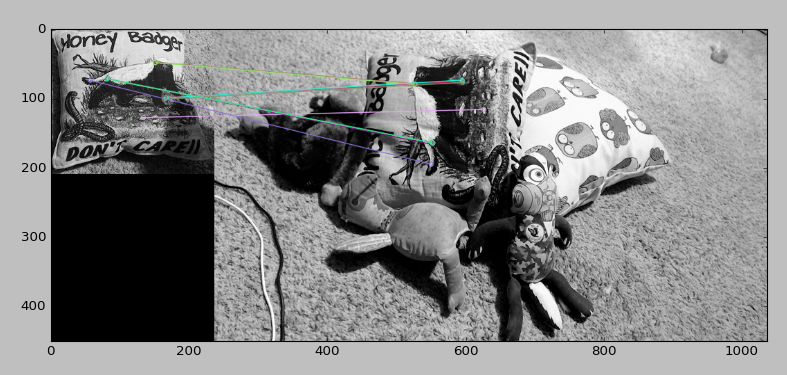## 十五、MOG 背景减弱

import numpy as np
import cv2

cap = cv2.VideoCapture('people-walking.mp4')
fgbg = cv2.createBackgroundSubtractorMOG2()

while(1):

k = cv2.waitKey(30) & 0xff
if k == 27:
break

cap.release()
cv2.destroyAllWindows()

https://pythonprogramming.net/static/images/opencv/opencv-python-foreground.mp4

import numpy as np
import cv2

cap = cv2.VideoCapture(0)

while 1:
gray = cv2.cvtColor(img, cv2.COLOR_BGR2GRAY)
faces = face_cascade.detectMultiScale(gray, 1.3, 5)

    for (x,y,w,h) in faces:
cv2.rectangle(img,(x,y),(x+w,y+h),(255,0,0),2)
roi_gray = gray[y:y+h, x:x+w]
roi_color = img[y:y+h, x:x+w]

        eyes = eye_cascade.detectMultiScale(roi_gray)
for (ex,ey,ew,eh) in eyes:
cv2.rectangle(roi_color,(ex,ey),(ex+ew,ey+eh),(0,255,0),2)

    cv2.imshow('img',img)
k = cv2.waitKey(30) & 0xff
if k == 27:
break

cap.release()
cv2.destroyAllWindows()

import numpy as np
import cv2

cap = cv2.VideoCapture(0)

while 1:
gray = cv2.cvtColor(img, cv2.COLOR_BGR2GRAY)

for (x,y,w,h) in faces:
cv2.rectangle(img,(x,y),(x+w,y+h),(255,0,0),2)
roi_gray = gray[y:y+h, x:x+w]
roi_color = img[y:y+h, x:x+w]

for (ex,ey,ew,eh) in eyes:
cv2.rectangle(roi_color,(ex,ey),(ex+ew,ey+eh),(0,255,0),2)

cv2.imshow('img',img)
k = cv2.waitKey(30) & 0xff
if k == 27:
break

cap.release()
cv2.destroyAllWindows()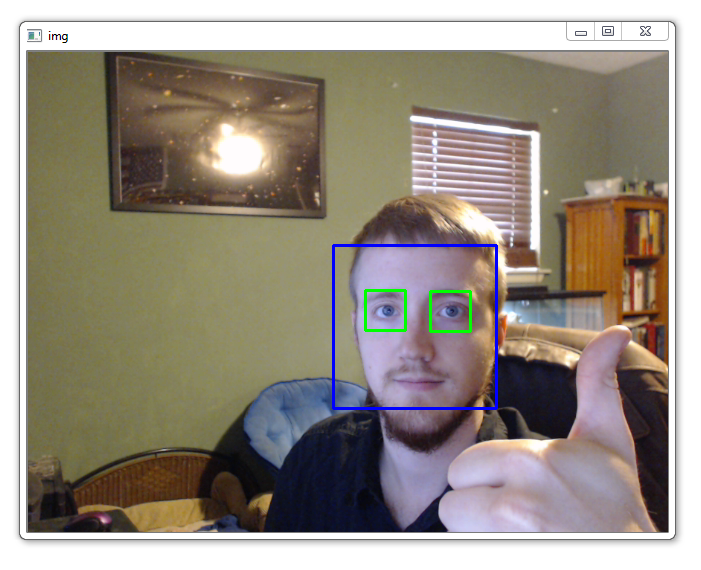cd ~

sudo apt-get update

sudo apt-get upgrade

mkdir opencv_workspace

cd opencv_workspace

sudo apt-get install git

git clone https://github.com/Itseez/opencv.git

Python 绑定：sudo apt-get install python-dev python-numpy libtbb2 libtbb-dev libjpeg-dev libpng-dev libtiff-dev libjasper-dev libdc1394-22-dev

sudo apt-get install libopencv-dev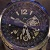# download-image-by-link.py

import urllib.request
import cv2
import numpy as np
import os

def store_raw_images():
pic_num = 1

if not os.path.exists('neg'):
os.makedirs('neg')

for i in neg_image_urls.split('\n'):
try:
print(i)
urllib.request.urlretrieve(i, "neg/"+str(pic_num)+".jpg")
# should be larger than samples / pos pic (so we can place our image on it)
resized_image = cv2.resize(img, (100, 100))
cv2.imwrite("neg/"+str(pic_num)+".jpg",resized_image)
pic_num += 1

except Exception as e:
print(str(e))  

def find_uglies():
match = False
for file_type in ['neg']:
for img in os.listdir(file_type):
for ugly in os.listdir('uglies'):
try:
current_image_path = str(file_type)+'/'+str(img)
if ugly.shape == question.shape and not(np.bitwise_xor(ugly,question).any()):
print('That is one ugly pic! Deleting!')
print(current_image_path)
os.remove(current_image_path)
except Exception as e:
print(str(e))

def store_raw_images():
pic_num = 953

if not os.path.exists('neg'):
os.makedirs('neg')

for i in neg_image_urls.split('\n'):
try:
print(i)
urllib.request.urlretrieve(i, "neg/"+str(pic_num)+".jpg")
# should be larger than samples / pos pic (so we can place our image on it)
resized_image = cv2.resize(img, (100, 100))
cv2.imwrite("neg/"+str(pic_num)+".jpg",resized_image)
pic_num += 1

except Exception as e:
print(str(e)) 

def create_pos_n_neg():
for file_type in ['neg']:

for img in os.listdir(file_type):

if file_type == 'pos':
line = file_type+'/'+img+' 1 0 0 50 50\n'
with open('info.dat','a') as f:
f.write(line)
elif file_type == 'neg':
line = file_type+'/'+img+'\n'
with open('bg.txt','a') as f:
f.write(line)

opencv_workspace
--neg
----negimages.jpg
--opencv
--info
--bg.txt
--watch5050.jpg

opencv_createsamples -img watch5050.jpg -bg bg.txt -info info/info.lst -pngoutput info -maxxangle 0.5 -maxyangle 0.5 -maxzangle 0.5 -num 1950


0001_0014_0045_0028_0028.jpg 1 14 45 28 28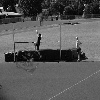opencv_createsamples -info info/info.lst -num 1950 -w 20 -h 20 -vec positives.vec

mkdir data，你的工作空间应该如下所示：

opencv_workspace
--neg
----negimages.jpg
--opencv
--info
--data
--positives.vec --bg.txt
--watch5050.jpg

opencv_traincascade -data data -vec positives.vec -bg bg.txt -numPos 1800 -numNeg 900 -numStages 10 -w 20 -h 20

nohup opencv_traincascade -data data -vec positives.vec -bg bg.txt -numPos 1800 -numNeg 900 -numStages 10 -w 20 -h 20 &

import numpy as np
import cv2

cap = cv2.VideoCapture(0)

while 1:
gray = cv2.cvtColor(img, cv2.COLOR_BGR2GRAY)

# image, reject levels level weights.

for (x,y,w,h) in watches:
cv2.rectangle(img,(x,y),(x+w,y+h),(255,255,0),2)

for (x,y,w,h) in faces:
cv2.rectangle(img,(x,y),(x+w,y+h),(255,0,0),2)

roi_gray = gray[y:y+h, x:x+w]
roi_color = img[y:y+h, x:x+w]
for (ex,ey,ew,eh) in eyes:
cv2.rectangle(roi_color,(ex,ey),(ex+ew,ey+eh),(0,255,0),2)

cv2.imshow('img',img)
k = cv2.waitKey(30) & 0xff
if k == 27:
break

cap.release()
cv2.destroyAllWindows()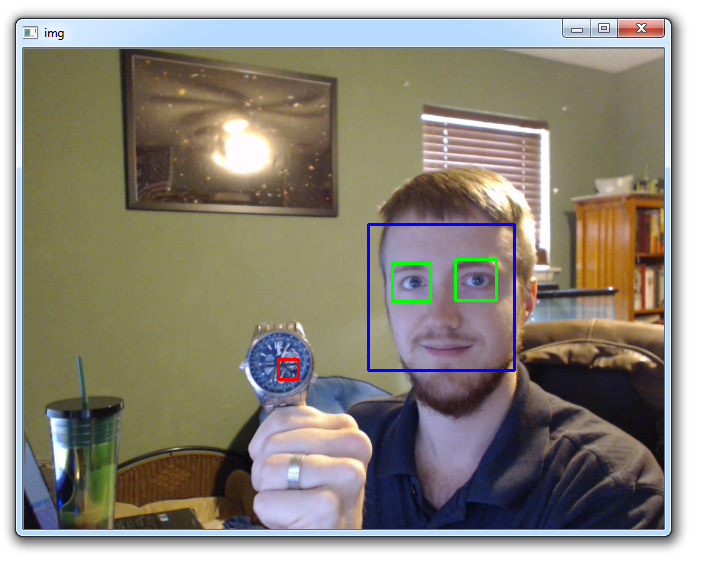font = cv2.FONT_HERSHEY_SIMPLEX
cv2.putText(img,'Watch',(x-w,y-h), font, 0.5, (11,255,255), 2, cv2.LINE_AA)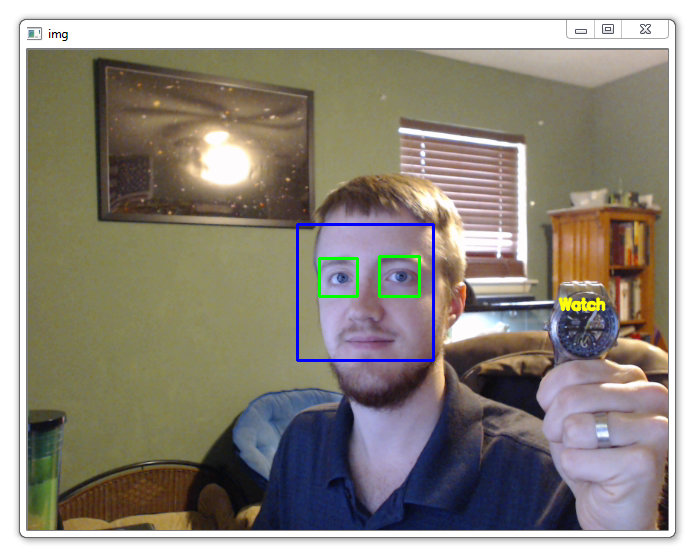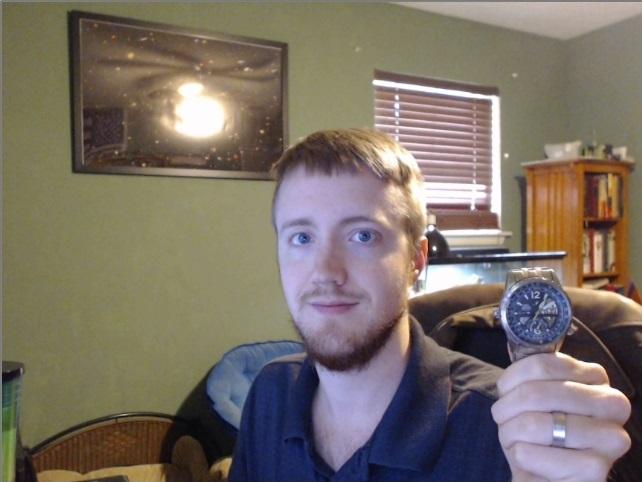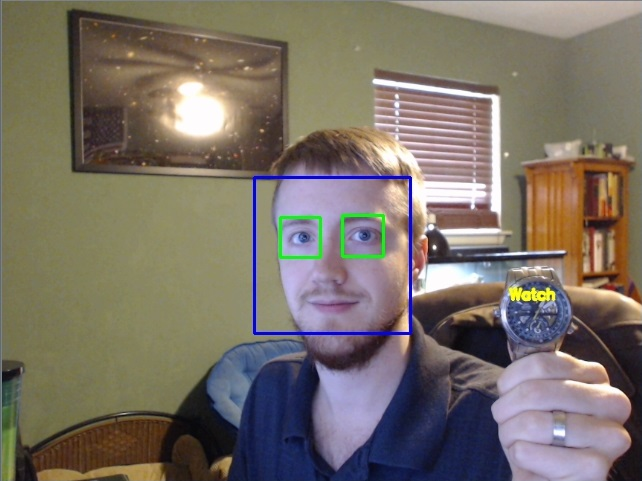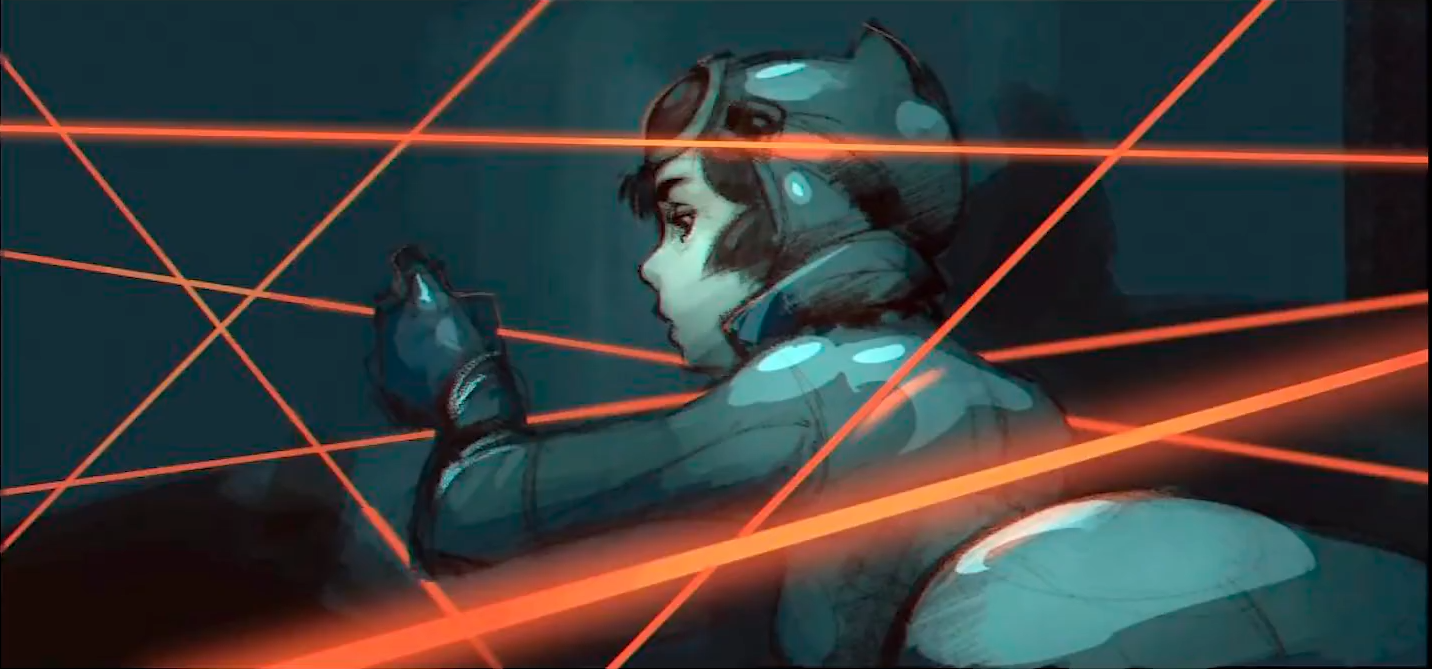OpenCV将两幅图像拼在一起（Python）
OpenCV将两幅图像拼在一起（Python）
79 0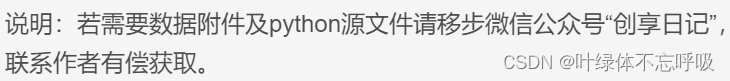Python计算细胞核与细胞质的面积比opencv或pil实验
Python计算细胞核与细胞质的面积比opencv或pil实验
85 0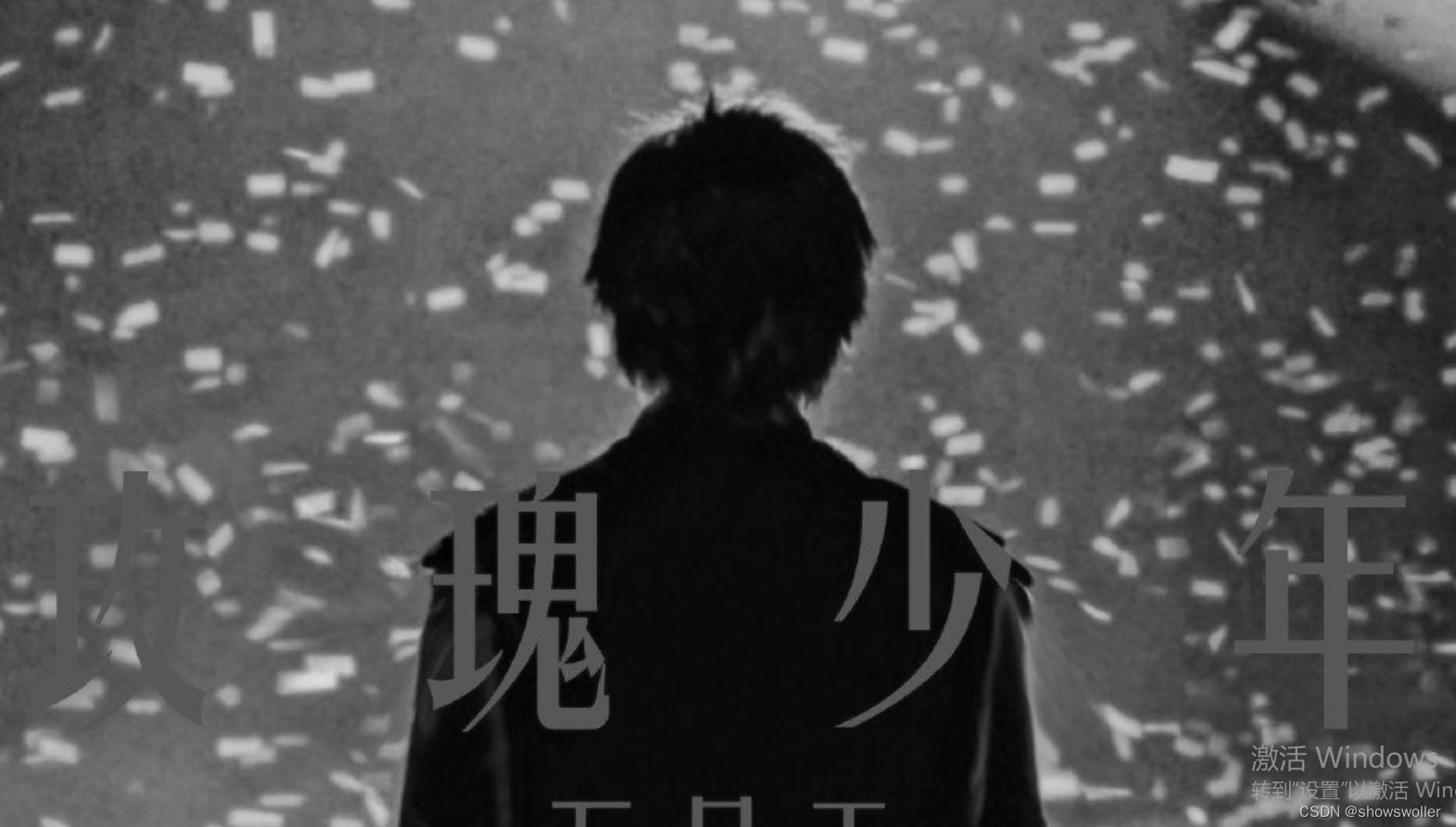python机器学习入门之opencv的使用（超详细，必看）
python机器学习入门之opencv的使用（超详细，必看）
125 0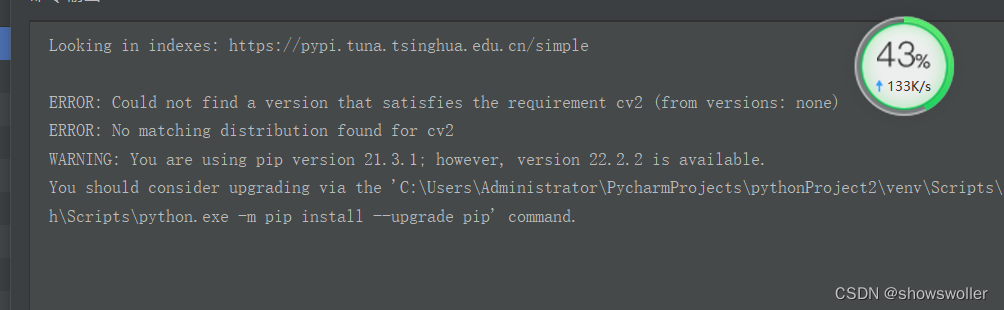python机器学习入门之opencv的安装与环境配置（超详细，避坑必看，包括没代码提示的解决）
python机器学习入门之opencv的安装与环境配置（超详细，避坑必看，包括没代码提示的解决）
165 0Python 基于OpenCV+face_recognition实现人脸
q
47 0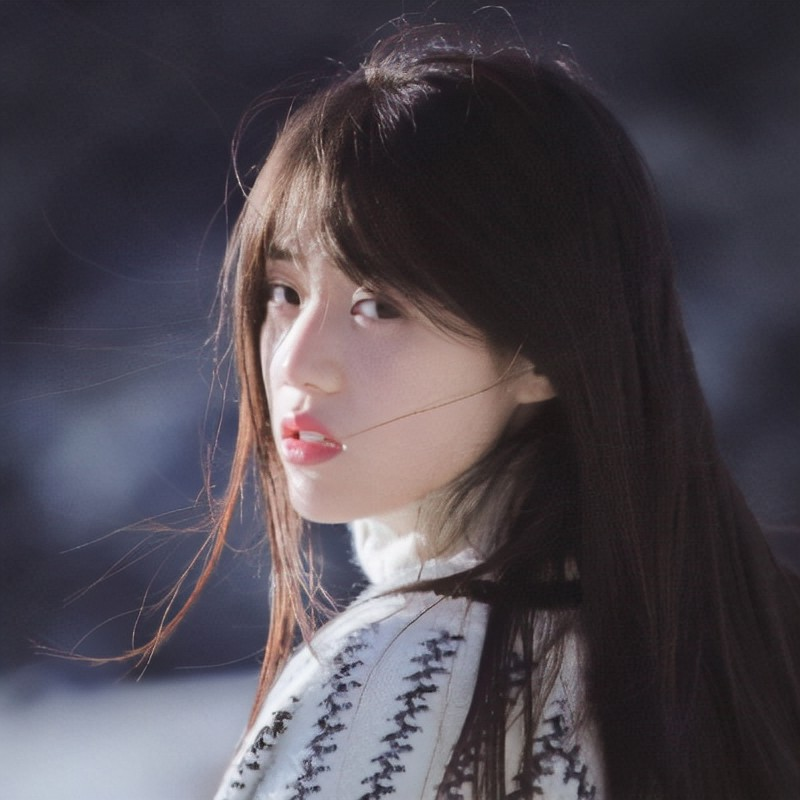## 介绍： 作为初学者，当您开始使用OpenCV时，有必要了解一些几乎每次都会在opencv项目中使用的方法。下面列出的是OpenCV中最常用的五种方法（请记住OpenCV作为cv2导入）
81 0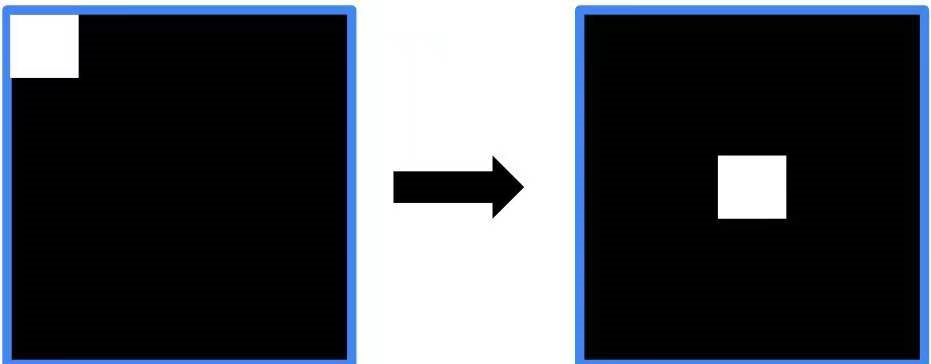opencv 傅里叶变换(python)
opencv 傅里叶变换(python)
160 0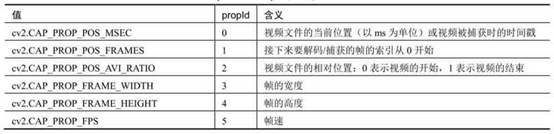opencv 视频处理(python)
opencv 视频处理(python)
302 0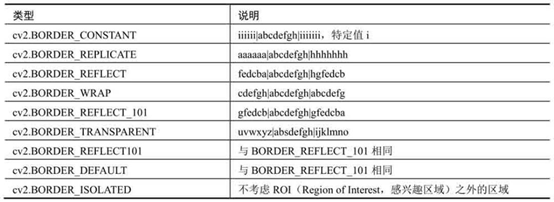opencv 形态学操作(python)
opencv 形态学操作(python)
107 0

Github：@wizardforcel 简书：@ApacheCN_飞龙 微博：@龙雀 CSDN：@wizardforcel ApacheCN 官网：apachecn.org 机器学习交流群：629470233

Python基础快速入门实战教程377908